Courses
Courses for Kids
Free study material
Free LIVE classes
MoreLIVE
Join Vedantu’s FREE Mastercalss

## Coordination Compounds Revision Notes Free PDF for NEET Preparation

The Class 12 NEET syllabus covers the topic of coordination compounds in an elaborate way. This chapter explains how metals form a different type of electronic bond where the atoms donate a pair of electrons to an empty shell or an atom or a functional group. It will explain how different compounds form with such bonds. To understand the concepts of this chapter, refer to the Coordination Compounds notes.

These revision notes are designed by the subject experts of Vedantu to offer a simpler explanation of all the fundamental principles of the coordination compounds. It will become a lot easier for the NEET aspirants to go through the chapter faster using these notes.

Last updated date: 23rd Sep 2023
Total views: 128.1k
Views today: 3.28k

## Access NEET Revision Notes Chemistry Coordination Compounds

### Introduction

Coordination compounds have a crucial role. It is important to understand that without the existence of hemoglobin (a Fe-complex found in human blood) and chlorophyll (a Mg-complex found in plants), life would not have been conceivable. Our understanding of chemical bonding and the physical characteristics of coordination compounds, such as their magnetic properties, will be expanded through the study of these compounds.

When a solution containing two or more simple stable compounds in molecular proportions is allowed to combine, it forms crystals of new substances known as addition compounds.

Example:

$\begin{array}{l}{\rm{KCl \ + \ MgC}}{{\rm{l}}_{\rm{2}}}{\rm{ \ + \ 6}}{{\rm{H}}_{\rm{2}}}{\rm{O }} \to {\rm{ }}\mathop {{\rm{KCl}}{\rm{.MgC}}{{\rm{l}}_{\rm{2}}}.6{{\rm{H}}_{\rm{2}}}{\rm{O}}}\limits_{\left( {{\rm{Carnallite}}} \right)} \\{\rm{CuS}}{{\rm{O}}_{\rm{4}}}{\rm{ \ + \ 4N}}{{\rm{H}}_{\rm{3}}}{\rm{ }} \to {\rm{ }}\mathop {\left[ {{\rm{Cu}}{{\left( {{\rm{N}}{{\rm{H}}_3}} \right)}_4}} \right]{\rm{S}}{{\rm{O}}_4}}\limits_{\left( {{\rm{Tetrammine copper }}\left( {{\rm{II}}} \right){\rm{ sulphate}}} \right)} \end{array}$

### 1. Double Salt

When two different salts are combined, a compound known as a "double salt" is created. This substance crystallises as a single substance but, when dissolved in water, ionises as two different salts. These salts undergo salt identity loss in solution, which causes them to test positive for all of the salt's ions when dissolved in water. such as Mohr's salt and potash alum.

Example:

$\mathop {{\rm{FeS}}{{\rm{O}}_{\rm{4}}}{\rm{.}}{{\left( {{\rm{N}}{{\rm{H}}_{\rm{4}}}} \right)}_{\rm{2}}}{\rm{S}}{{\rm{O}}_{\rm{4}}}{\rm{.6}}{{\rm{H}}_{\rm{2}}}{\rm{O }}}\limits_{{\rm{Mohr's salt }}} \to {\rm{ F}}{{\rm{e}}^{{\rm{2 \ + \ }}}}_{\left( {aq} \right)}{\rm{ \ + \ 6}}{{\rm{H}}_{\rm{2}}}{\rm{O \ + \ 2N}}{{\rm{H}}_{\rm{4}}}{^{\rm{ \ + \ }}_{\left( {aq} \right)}}{\rm{ \ + \ 2S}}{{\rm{O}}_{\rm{4}}}{^{{\rm{2-}}}_{\left( {aq} \right)}}$

### 2. Coordination Compounds

A coordination compound is made up of a ligand, a central atom, a complex ion, a cation, or an anion. The ion (cation or anion) is typically written outside the complex ion (which is typically represented in a square box).

eg: $\begin{array}{l}\left[ {{\rm{Co}}{{\left( {{\rm{N}}{{\rm{H}}_{\rm{3}}}} \right)}_{\rm{6}}}{\rm{ }}} \right]{\rm{ C}}{{\rm{l}}_{\rm{3}}}{\rm{ }}\\\left[ {{\rm{Complex~ion}}} \right]{\rm{ anion}}\end{array}$

General formula: ${{\rm{A}}_{\rm{x}}}\left[ {{\rm{M}}{{\rm{L}}_{\rm{n}}}} \right]{\rm{/}}\left[ {{\rm{M}}{{\rm{L}}_{\rm{n}}}} \right]{{\rm{B}}_{\rm{y}}}$  where M is the central metal atom/ion, L is the ligand, A is the cation and B is the anion.

### Coordination Entity

It is the fixed central metal atom or ion that is bonded to a specific number of ions or molecules. For example, six ammonia molecules are bonded with coordination bonds to Cu2+ which are surrounded by three chloride ions in $\left[ {{\rm{Co}}{{\left( {{\rm{N}}{{\rm{H}}_{\rm{3}}}} \right)}_{\rm{6}}}} \right]{\rm{C}}{{\rm{l}}_{\rm{3}}}{\rm{,}}$  a coordination entity.

### Central Atom/ion

In a specific geometrical arrangement, it is the central cation that is surrounded and coordinately bonded to one or more neutral molecules or negatively charged ions. In the complex $\left[ {{\rm{Co}}{{\left( {{\rm{N}}{{\rm{H}}_{\rm{3}}}} \right)}_{\rm{6}}}} \right]{\rm{C}}{{\rm{l}}_{\rm{3}}}{\rm{,}}$  for example, ${\rm{C}}{{\rm{o}}^{{\rm{3 + }}}}$  is the central metal ion that is positively charged and is coordinately bonded to six neutral NH3 molecules within the coordination sphere. The central metal/ion is also known as Lewis acid.

### Ligands

Ligands are ions or molecules that are joined to the main atom/ion of the coordination entity. These can be simple ions like ${\rm{C}}{{\rm{l}}^{\rm{-}}}{\rm{,}}$  small molecules like ${{\rm{H}}_{\rm{2}}}{\rm{O~or~N}}{{\rm{H}}_{\rm{3}}}{\rm{,}}$  or larger molecules like ${{\rm{H}}_{\rm{2}}}{\rm{NC}}{{\rm{H}}_{\rm{2}}}{\rm{C}}{{\rm{H}}_{\rm{2}}}{\rm{N}}{{\rm{H}}_{\rm{2}}}{\rm{.}}$

### Coordination Number (C.N)

The number of atoms in the ligands that are directly bound to the central metal atom or ion by coordinate bonds is known as the metal atom's or ion's coordination number. It is also the same as secondary valency.

$\begin{array}{*{20}{l}}{{\rm{Complex}}}&{{\rm{Coordination\, number}}}\\{{{\rm{K}}_{\rm{4}}}\left[ {{\rm{Fe}}{{\left( {{\rm{CN}}} \right)}_{\rm{6}}}} \right]}&{\rm{6}}\\{{{\left[ {{\rm{Ag}}{{\left( {{\rm{CN}}} \right)}_{\rm{2}}}} \right]}^ - }}&{\rm{2}}\\{\left[ {{\rm{Pt}}{{\left( {{\rm{N}}{{\rm{H}}_{\rm{3}}}} \right)}_{\rm{2}}}{\rm{C}}{{\rm{l}}_{\rm{2}}}} \right]}&{\rm{4}}\\{{{\left[ {{\rm{Ca}}\left( {{\rm{EDTA}}} \right)} \right]}^{2 - }}}&{\rm{6}}\end{array}$

### Coordination Sphere

The ligands are directly bonded to the core metal atom or ion are enclosed in a square bracket. The first sphere of attraction or the coordination sphere was referred to as the Coordination sphere. The coordination sphere of the metal ion closely encloses the ligands, causing it to function as a single entity.

### Co-ordination Polyhedron

The spatial arrangement of the ligand atoms that are directly attached to the central atom/ion is referred to as a coordination polyhedron. For example, ${\left[ {{\rm{Co}}{{\left( {{\rm{N}}{{\rm{H}}_{\rm{3}}}} \right)}_{\rm{6}}}} \right]^{{\rm{3 + }}}}{\rm{ }}$  is octahedral, $\left[ {{\rm{Ni}}{{\left( {{\rm{CO}}} \right)}_{\rm{4}}}} \right]$  is tetrahedral, and ${\left[ {{\rm{PtC}}{{\rm{l}}_{\rm{4}}}} \right]_{\rm{2}}}$  is square planar.

### Oxidation Number of Central Metal Atom

It is defined as the charge that the central metal ion would have if all ligands and electron pairs were removed. It is computed as follows:

Example:

${{\rm{K}}_{\rm{4}}}\left[ {{\rm{Fe}}{{\left( {{\rm{CN}}} \right)}_{\rm{6}}}} \right] \to 4{{\rm{K}}^ + } + {\left[ {{\rm{Fe}}{{\left( {{\rm{CN}}} \right)}_{\rm{6}}}} \right]^{4 - }}$

Charge on complex ion is -4.

Let charge on Fe be x.

Now, charge on cyanide ion is -1.

$\begin{array}{l} \Rightarrow x + 6 \times \left( { - 1} \right) = - 4\\ \Rightarrow x = + 2\end{array}$

Hence, the oxidation number of Fe is +2 (II).

### Homoleptic and Heteroleptic Complexes

Homoleptic complexes are those in which the central atom is coordinated with only one type of ligand, such as ${\left[ {{\rm{Co}}{{\left( {{\rm{N}}{{\rm{H}}_{\rm{3}}}} \right)}_6}} \right]^{{\rm{3 + }}}}{\rm{.}}$  Hetroleptic complexes are those in which the central atom is coordinated with more than one type of ligand, such as ${\left[ {{\rm{Co}}{{\left( {{\rm{N}}{{\rm{H}}_{\rm{3}}}} \right)}_4}{\rm{C}}{{\rm{l}}_2}} \right]^{\rm{ + }}}{\rm{.}}$

### Nomenclature

The following rules are followed when naming a complex ion:

(a) Cations are named first, followed by anions.

(b) The central metal ion's oxidation state (O.S.) is denoted by a Roman numeral.

(c) The ligand names are listed first, followed by the name of the central metal ion.

(d) Anion ligand names that end in 'ide' are changed to 'o', 'ite' are changed to 'ito' and 'ate' are changed to 'ato'

(e) The unmodified name is used for many ligands that are molecules.

(f) Positive groups are terminated by –ium. For example: $\mathop {\rm{N}}\limits^{ \cdot \cdot } {{\rm{H}}_2} - {\rm{N}}{{\rm{H}}_3}^ +$  hydrazinium.

(g) When there are multiple ligands of the same type, the prefixes di, tri, tetra, penta, and hexa are used to indicate the number of ligands of that type. An exception occurs when the name of the ligand contains a number, as in ethylenediamine (en). To avoid confusion, bis, tris, and tetrakis are used instead of di, tri, and tetra, and the ligand name is enclosed in brackets. as in bis (ethylenediamine)

(h) If anion is a complex, metal is followed by 'ate'.

${\left[ {{\rm{Ni}}{{\left( {{\rm{CN}}} \right)}_4}} \right]^{2 - }}$ : tetracyanonickelate (II) ion

gold – aurate

zinc – zincate

tin – stannate

silver – argentate

cobalt – cobaltate

iron – ferrate

aluminium – aluminate

manganese – manganate

copper – cuprate

chromium – chromate

platinum – platinate

(i) A complex is said to be polynuclear if it contains two or more metal atoms. The prefix – $\mu$ denotes the bridging ligands that connect the two metal atoms.

(j) Ambidentate ligands can be connected via different atoms.–

$\begin{array}{l}{\rm{M}} \leftarrow {\rm{N}}{{\rm{O}}_{\rm{2}}}\\{\rm{M}} \leftarrow {\rm{ONO}}\end{array}$

(k) The complex formula should be written (not named) as follows:

• Complex ions should be contained in square brackets and

• Ligands should be listed alphabetically after metal.

### Werner’s Theory

Werner concluded that the metal in complexes had two distinct forms of valency after describing the nature of bonding in complexes.

### 1. Primary Valency

The oxidation state of the central metal atom or ion determines the primary valency. These are asymmetrical.

Example: What are the primary valency of ${{\rm{K}}_4}\left[ {{\rm{Fe}}{{\left( {{\rm{CN}}} \right)}_{\rm{6}}}} \right]{\rm{ \& }}\left[ {{\rm{Cu}}{{\left( {{\rm{N}}{{\rm{H}}_{\rm{3}}}} \right)}_{\rm{4}}}} \right]{\rm{S}}{{\rm{O}}_{\rm{4}}}$ ?

Sol. The primary valency of ${{\rm{K}}_4}\left[ {{\rm{Fe}}{{\left( {{\rm{CN}}} \right)}_{\rm{6}}}} \right]{\rm{ \& }}\left[ {{\rm{Cu}}{{\left( {{\rm{N}}{{\rm{H}}_{\rm{3}}}} \right)}_{\rm{4}}}} \right]{\rm{S}}{{\rm{O}}_{\rm{4}}}$  is 2.

### 2. Secondary Valency

Secondary valency refers to the number of ligand atoms that are coordinated to the central metal atom. Because these are directional, a complex ion has a specific shape.

Example: What are the secondary valency of $\left[ {{\rm{Co}}{{\left( {{\rm{N}}{{\rm{H}}_{\rm{3}}}} \right)}_{\rm{6}}}} \right]{\rm{C}}{{\rm{l}}_{\rm{3}}}{\rm{ \& }}{{\rm{K}}_{\rm{4}}}\left[ {{\rm{Fe}}{{\left( {{\rm{CN}}} \right)}_{\rm{6}}}} \right]$  ?

Ans: The secondary valency in $\left[ {{\rm{Co}}{{\left( {{\rm{N}}{{\rm{H}}_{\rm{3}}}} \right)}_{\rm{6}}}} \right]{\rm{C}}{{\rm{l}}_{\rm{3}}}$  is 6.

${{\rm{K}}_{\rm{4}}}\left[ {{\rm{Fe}}{{\left( {{\rm{CN}}} \right)}_{\rm{6}}}} \right]$ : Six ligands are coordinated to Fe. The secondary valency is therefore 6. Primary valency is satisfied by ions that are joined to complex ions. Dotted lines are used to depict it. Primary valency is also known as ionisable valency. The ligands satisfy the secondary valency; they are non-ionisable and are represented by a solid line $\left[ {{\rm{Co}}{{\left( {{\rm{N}}{{\rm{H}}_{\rm{3}}}} \right)}_{\rm{6}}}} \right]{\rm{C}}{{\rm{l}}_{\rm{3}}}$Structure of Coordination Compound

Every element is capable of satisfying both its primary and secondary valencies. When a negative ion is present in the coordination sphere, it exhibits dual behavior. It has the potential to satisfy both primary and secondary valencies.

The ligands that satisfy the secondary valencies are aimed at specific locations in space. The coordination number determines the geometry of the complex ion. If the metal has coordination number 6, the complex is octahedral, which means that six donor atoms of the ligands occupy six positions around the metal octahedrally. If, on the other hand, the coordination number is 4, the complex's geometry can be tetrahedral or square planar. This postulate predicted that different types of isomerism would exist in coordination compounds.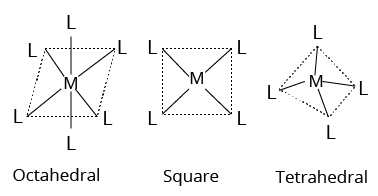Different Types of Isomerism Exist in Coordination Compound

Familiar C.N.’s of some common metal ions.

 Univalent C.N Divalent C.N. Ag+ 2 V2+ 6 Au+ 2, 4 Fe2+ 6 Ti+ 2 Co2+ 4, 6 Cu+ 2, 4 Ni2+ 4, 6 Cu2+ 4, 6 Zn2+ 4 Pd2+ 4 Pt2+ 4 Ag2+ 4

 Trivalent C.N. Tetravalent C.N. Sc3+ 6 Pt4+ 6 Cr3+ 6 Pd4+ 6 Fe2+ 6 Co3+ 6 Os3+ 6 Ir3+ 6 Au3+ 4

### Effective Atomic Number (EAN)

Effective atomic number (EAN), which Sidgwick proposed, is the quantity of electrons that a metal atom or ion has after receiving them from the donor atoms of its ligands. In some circumstances, the effective atomic number (EAN) and the atomic number of the nearest inert gas are the same. The formula for calculating EAN is as follows:

EAN = Atomic number of the metal – number of electrons lost in ion formation + number of electrons gained from the donor atoms of the ligands.

The EAN values of various metals in their complexes are listed below:

 Complex Metal (Oxidation state) Atomic Number of Metal Coordination Number Effective Atomic Number ${{\rm{K}}_{\rm{4}}}\left[ {{\rm{Fe}}{{\left( {{\rm{CN}}} \right)}_{\rm{6}}}} \right]$ +2 26 6 $\left( {26 - 2} \right) + \left( {6 \times 2} \right) = 36$ $\left[ {{\rm{Cu}}{{\left( {{\rm{N}}{{\rm{H}}_{\rm{3}}}} \right)}_{\rm{4}}}} \right]{\rm{S}}{{\rm{O}}_{\rm{4}}}$ +2 29 4 $\left( {29 - 2} \right) + \left( {4 \times 2} \right) = 35$ $\left[ {{\rm{Co}}{{\left( {{\rm{N}}{{\rm{H}}_{\rm{3}}}} \right)}_{\rm{6}}}} \right]{\rm{C}}{{\rm{l}}_{\rm{4}}}$ +3 27 6 $\left( {27 - 3} \right) + \left( {6 \times 2} \right) = 36$ ${\rm{Ni}}{\left( {{\rm{CO}}} \right)_{\rm{4}}}$ 0 28 4 $\left( {28 - 0} \right) + \left( {4 \times 2} \right) = 36$ ${{\rm{K}}_{\rm{2}}}\left[ {{\rm{Ni}}{{\left( {{\rm{CN}}} \right)}_{\rm{4}}}} \right]$ +2 28 4 $\left( {28 - 2} \right) + \left( {4 \times 2} \right) = 34$

### Valence Bond Theory

Valence Bond Theory (VBT) can explain the bonding in coordination compounds because the d orbitals of the majority of transition metal complexes are incomplete. Valence bond considers orbital hybridization because penultimate d-orbitals are close in energy to s and p-orbitals of the outermost shell, allowing for various types of hybridization.

The following assumption is made by VBT:

(i) The central metal ion has a number of empty orbitals that can accept electrons donated by the ligands. The coordination number of the metal ion for the specific complex is equal to the number of empty s, p, d-orbitals, .

(ii) Strong bonds are formed when the metal orbitals and ligand orbitals overlap. The greater the extent of overlapping, the more stable the complex. Different orbitals (s, p, or d) hybridize to form a set of equivalent hybridized orbitals that participate in ligand bonding.

(iii) Generally, each ligand contributes two electrons to the central metal ion/atom.

(iv) The inner orbitals contain non-bonding metal electrons that do not participate in chemical bonding.

(v) A complex is paramagnetic if it contains unpaired electrons. The complex is diamagnetic if it does not contain an unpaired electron.

(vi) Under the influence of a strong ligand (CN, CO), electrons can be forced to pair up, thereby violating Hund's rule of multiplicity.

### Common Types of Hybridization

 Coordination Number Hybridization Shape Geometry 2 $sp$ Linear ${\rm{X}} - {\rm{A}} - {\rm{X}}$ 4 $s{p^3}$ Tetrahedral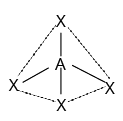4 $ds{p^2}$ Square planar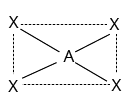5 $\begin{array}{l}ds{p^3}\\{\rm{or \ }}s{p^3}d\end{array}$ TrigonalBipyramidal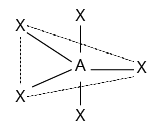6 $\begin{array}{l}{d^2}s{p^3}\\{\rm{or \ }}s{p^3}{d^2}\end{array}$ OctahedralNote:

Inner d-orbitals (3d orbital) have been used for bonding in d2sp3 hybridization; such complexes are known as inner orbital complexes or low spin complexes. The outer d-orbitals (4d orbital) have been used for bonding in sp3d2 hybridization; such complexes are known as outer orbital complexes or high spin complexes. $\sqrt {n\left( {n + 2} \right)}$  where n is the number of unpaired electrons, gives the magnetic moment.

### Limitations of VBT

1. The change in ligand and metal ion properties could not be explained.

2. The valence bond theory is silent on why some complexes are more labile than others.

3. The VBT does not explain the existence of inner and outer orbital complexes satisfactorily.

4. The VBT was unable to explain the color of complexes.

### Crystal Field Theory

Compared to the Crystal Field Theory, the Valence Bond Theory is less widely recognised. It is thought that the bonding between the ligands and the core metal of a complex is solely electrostatic. The crystal field operates under the presumptions listed below.

1. Ligands are considered point charges in case of anions or point dipoles in case of neutral molecules.

2. In the free atom, all of the d orbitals on the metal have the same energy (that is, they are degenerate).

However, when a complex is formed, the ligands destroy the degeneracy of these orbitals, resulting in different energies for the orbitals.

### Octahedral Complexes

The ligands are located at the six corners of an octahedral complex, which has the metal at its centre. The octahedron's three adjacent corners are indicated by the directions x, y, and z, respectively. The lobes of the ${{\rm{e}}_g}{\rm{ and }}{{\rm{d}}_{{{\rm{x}}^{\rm{2}}}{\rm{ - }}{{\rm{y}}^{\rm{2}}}}}{\rm{, }}{{\rm{d}}_{{{\rm{z}}^2}}}$  orbitals point along the x, y, and z axes and the lobes of the ${{\rm{t}}_{2g}}{\rm{~and~}}{{\rm{d}}_{{\rm{xy}}}}{\rm{, }}{{\rm{d}}_{{\rm{xz}}}}{\rm{, }}{{\rm{d}}_{{\rm{yz}}}}$ are located between the axes. The approach of six ligands along the x, y, z, –x, –y, and –z directions increases the energy of the ${{\rm{d}}_{{{\rm{x}}^{\rm{2}}}{\rm{ - }}{{\rm{y}}^{\rm{2}}}}}{\rm{~and~}}{{\rm{d}}_{{{\rm{z}}^2}}}$  orbitals (which point along the axes) much more than the energy of the dxy, dxz, and dyz orbitals (which point between the axes). Thus, the d orbitals split into two groups under the influence of an octahedral ligand field.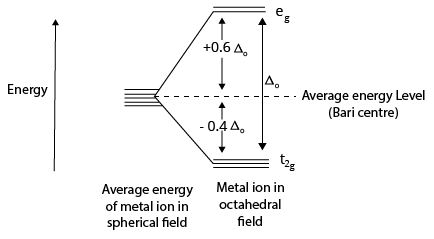Crystal Field Splitting Diagram

Weak field ligands are those that only slightly break the crystal field. Strong field ligands are those that result in a significant splitting. It is possible to arrange the common ligands in ascending crystal field splitting. $\Delta .$

### Spectrochemical Series

I < Br < S2– < Cl < NO3- < F < OH < EtOH < oxalate < H2

(weak field ligands)

< EDTA < (NH3 = pyridine) < ethylenediamine < dipyridyl < o- phenanthroline < NO2 < CN < CO  (strong field ligands)

A pattern of increasing donation is followed :

Halide donors < O donors < N donors < C donors

The total crystal field stabilization energy is given by

${\rm{CFS}}{{\rm{E}}_{\left( {{\rm{octahedral}}} \right)}} = - 0.4{n_{\left( {{t_{2g}}} \right)}} + 0.6{n_{\left( {{e_g}} \right)}}$

where ${n_{{t_{2g}}}}{\rm{ and }}{n_{{e_g}}}$  are the number of electrons occupying the t2g and eg orbitals respectively. The CFSE is zero for ions with d0 and d10 configurations in both strong and weak ligand fields. The CFSE is also zero for d5 configurations in a weak field.

### Tetrahedral Complexes

A cube is related to a regular tetrahedron. As shown, one atom is in the center of the cube, and four of the cube's eight corners are occupied by ligands.Tetrahedron Structure

The cube's face centres are indicated by the directions x, y, and z. The x, y, and z axes are where the e orbitals are oriented (that is to the centres of the faces). Between the x, y, and z axes are where the t2 orbitals are (that is towards the centres of the edges of the cube). The e or t2 orbitals are not perfectly in line with the ligands' approach directions.

The t2 orbitals are therefore more in line with the ligand direction than the e orbitals. The energy of both sets of orbitals increases as the ligands approach. The t2 orbitals' energy is enhanced the most since they are near the ligands. The opposite of that is true of the crystal field splitting in octahedral complexes.

The t2 orbitals are $0.4{\Delta _t}$   higher than the weighted average energy of the two groups (the Bari center), while the e orbitals are $0.6{\Delta _t}$  lower.

In tetrahedral complexes, the magnitude of the crystal field splitting is much smaller than in octahedral fields. This is due to two factors:

1. Because there are only four ligands rather than six, the ligand field is only two-thirds the size; consequently, the ligand field splitting is also two-thirds the size.

2. The orbital direction does not coincide with the ligand direction. This reduces the crystal field splitting by about two-thirds.

Thus the tetrahedral crystal field splitting ${\Delta _t}$  is roughly 2/3 × 2/3 = 4/9 of the octahedral crystal field splitting ${\Delta _t}.$

### 1. Analytical chemistry:

The analytical applications of coordination chemistry are in

(A) Qualitative and Quantitative Analysis:

Metal ions interact with a range of ligands to create coordinated compounds that are coloured. Metal ions are discovered via these processes. With the help of conventional or technological methods like gravimetry or colorimetry, coloured complexes can be used to evaluate metals. These are a few instances:

The addition of potassium ferrocyanide solution detects the presence of iron ions (Fe3+), resulting in the formation of Prussian blue complex.

(B) Volumetric Analysis:

Titration with EDTA can be used to determine the hardness of water. Ca2+ and Mg2+, the metal ions that cause hardness, form stable complexes with EDTA.

### 2. Metal Extraction and Purification:

By creating cyanide compounds with the ore, water-soluble metals like silver and gold can be recovered. Pure gold can be recovered by adding zinc to the solution. By first creating and then breaking down their coordination molecules, metals can also be refined. For instance, it is possible to transform impure nickel into pure nickel following extraction by first turning it into nickel carbonyl and then decomposing it.

### 3. Catalysis:

(a) Critical business processes employ coordination chemical catalysts. For instance, (a) the Ziegler-Natta catalyst (TiCl4 and trialkyl aluminium) is utilised as a catalyst in the synthesis of polyethene.

(b) In the hydrogenation of alkenes, the Wilkinson catalyst - RhCl(PPh3)3 is used.

(c) Various rhodium complexes, such as [Rh(CO)2I2], [Rh(Cl)(CO)(PPh3)2], or [Rh(Cl)(CO)2]2 are used as catalysts in the Monsanto acetic acid process in the presence of CH3I, I2or HI as activator.

### 4. Electroplating:

Gold, silver, and copper coordination compounds are used as components in baths used for electroplating articles made of other metals with these metals. K[Ag(CN)2] is used as an electrolyte in silver plating; K[Au(CN)2] is used as an electrolyte in gold plating; and K3 [Cu(CN)4] is used as an electrolyte in copper plating.

### 5. Biological Importance:

Coordination complexes are important biological compounds. Chlorophyll, for example, is a Mg2+ complex. This green pigment is essential for photosynthesis in plants. Similarly, hemoglobin, the red pigment found in blood, is a Fe2+ coordination complex, and vitamin B12, an essential nutrient, is a Co3+ complex.

### 6. Medicinal Uses:

Complexing or chelating compounds, in which a coordination complex is created between the poisonous metal in excess metal and the complexing agent, are employed in the treatment of metal poisoning. For instance, lead toxicity is treated with EDTA. Lead is captured by EDTA when it is given intravenously into the bloodstream and forms a compound that is eliminated in the urine. Other heavy metal poisonings that can be treated similarly with chelation therapy include those caused by mercury, arsenic, aluminium, chromium, cobalt, manganese, nickel, selenium, zinc, tin, and thallium.

### 1. Centre of Coordination (Central atom/ion or Acceptor atom/ion):

The cation or neutral atom that one or more ligands (neutral molecules or anions) are coordinated to serves as the centre of coordination. To take the electron pairs given by the ligand's donor atom, the central atom/orbitals ion's must be vacant. This explains why transition metals with unoccupied d-orbitals easily form coordination compounds.

For example in the complexes [Ni(NH3)6]2+ and [Fe(CN)6]3- , Ni2+ and Fe3+ respectively are the central ions.

### 2. Ligands

An atom, ion, or molecule that can give the central atom at least two electrons in order to create a coordinate bond is known as a ligand or coordinating group (or dative linkage). The donor atom in a ligand is the one that really contributes the electron pair. The core metal atoms or ions serve as Lewis acids by absorbing electrons, while the ligands serve as Lewis bases by donating one or more electron pairs to them.

### Types of Ligands:

Ligands are classified based on how many lone pair electrons they donate to the central metal atom or ion.

(a) Monodentate or unidentate ligands: These ligands have a single donor atom that donates only one electron pair to the central metal atom.

(b) Bidentate ligands: Ligands with two donor atoms and the ability to link with the central metal in two positions are referred to as bidentate ligands.

(c) Tridentate Ligand: The ligands possess three donor atoms are called tridentate ligands

(d) Tetradentate ligand: These ligands having four donor atoms.

(e) Pentadentate ligands: They have five donor atoms

(f) Hexadentate Ligands: They have six donor atoms.

### 3. Chelating Ligands:

If a bidentate or polydentate ligand coordinates to create a cyclic ring structure, it is referred to as a chelating ligand. Complexes that come from this mechanism are called chelates. Chelates having rings of five or six members are more stable. Larger group ligands generate more unstable rings than smaller group ligands as a result of steric hindrance.

### 4. Ambidentate Ligands:

The ligands that have two donor atoms but only one donor atom is attached to the metal atom at a time when forming complexes. These ligands are known as ambidentate ligands. As an example:

$\begin{array}{cc}\mathrm{M} \leftarrow \mathrm{NO}_{2} & \mathrm{M} \leftarrow \mathrm{ONO} \\\text { Nitrito-N } & \text { Nitrito-O } \\\mathrm{M} \longleftarrow \mathrm{CN} & \mathrm{M} \leftarrow \mathrm{NC} \\\text { Cyano-C } & \text { Isocyano } \\\mathrm{M} \leftarrow \mathrm{SCN} & \mathrm{M} \leftarrow \mathrm{NCS} \\\text { Thiocyanato-S } & \text { Thiocyanato-N }\end{array}$

### Solved Examples:

1. A chelating agent has two or more than two donor atoms to bind to a single metal ion. Which of the following is not a chelating agent?

A: thiosulphato

B: oxalato

C: glycinato

D: ethane-1,2-diamine

Correct option: A

Explanation:

A chelating agent has two or more than two donor atoms to bind to a single metal ion. Oxaloto, Glycinato and ethane-1,2-diamine has 2 oxygen atoms that are donor atoms. Thiosulpato is an ambidentate ligand.

2. Which of the following species is not expected to be a ligand?

A: $NO$

B: $N{H^{4 + }}$

C: $N{H_2}C{H_2}C{H_2}N{H_2}$

D: $CO$

Correct option: B

Explanation:

Ligands donate electrons to metal ions via coordination bond. $N{H_4^{ + }}$ does not have a lone pair of electrons to give. Therefore, the correct option is B.

## Importance of NEET Class 12 Chemistry Coordination Compounds

This chapter explains how metal atoms form complex compounds with other functional groups. Two of the best examples of such compounds that can be found in the plant and animal kingdom are chlorophyll and haemoglobin, respectively. These compounds form a temporary bond for transporting important gases to their respective locations.

This chapter will explain a particular type of bonding that metal ions form with other atoms or groups of atoms due to the excess electrons present in the valence shells. According to the Coordination Compounds Class 12 notes, this type of chemical bond forms the additional compounds such as double salts.

The coordination compounds have the unique property of retaining the chemical features of the constituent cations, anions, and the complex ions present in their molecules. If you refer to the Coordination Compounds notes, you will find every coordination compound comprises a central metal atom that holds the integrity of the complex molecules.

The importance of this chapter lies in the definition, description, and formula of the coordination compounds. All the terms in this chapter will be explained with proper formulae and examples. It will also explain the structures of the molecules of such compounds.

All the theories and laws will be explained in this chapter using proper derivations and descriptions of the concepts related to primary valency, secondary valency, ligands, etc.

## Benefits of Vedantu’s Coordination Compounds Class 12 Notes for NEET

• All the concepts, definitions, derivations, and formulae of this chapter will be concisely discussed in the revision notes for NEET aspirants. Using these notes will help you understand the concepts faster.

• The easier format of this chapter will enable you to revise it faster. In fact, you will be able to remember what you have studied due to a clarified and organised presentation in the Coordination Compounds Class 12 notes for NEET.

• The easier format will also help you to recall what you have studied during the competitive exam. Students often find it tough to remember the fundamental principles accurately after studying the textbooks. Due to the intuitive presentation of all the concepts in these notes, you will be able to recall the right answers to all the fundamental questions asked in the chemistry section of the exam.

• The sample questions in the revision notes will enable you to evaluate your preparation level for this chapter. You will be able to find out which sections you need to study more and make your preparation better.

The free PDF version is now available for download. You can complete your study material for this chapter and proceed with the preparation. There is no need for you to struggle while making concise notes for this chapter when you can refer to expert-curated notes easily. Focus on your studies and refer to the precise Coordination Compounds NEET notes for quick revision.

## FAQs on Coordination Compounds Class 12 Notes NEET Chemistry [Free PDF Download]

1. What are isomers?

The compounds that have the same molecular formula but differ in their structural formula are called isomers.

2. What is crystal field theory?

According to this theory, the bond between the central metal atom and the ligands is electrostatic in nature.

3.  What is valence bond theory?

This theory explains that bonding occurs in the transition metals due to the incomplete d orbitals.

4. What are ligands?

The atoms, groups of atoms or ions attached to a central metal atom of a coordination compound are called ligands.